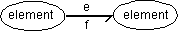﻿ 20-sim webhelp > Modeling Tutorial > Bond Graphs > Effort and Flow

# Effort and Flow

A bond between two elements transfers power from one element to the other. This flow of energy can be described in many ways. For bond graphs a uniform approach is chosen:

The flow of energy between two elements (and thus a bond) is always characterized by two variables, of which the product is power. According to the bond graph notation, these variables are called effort (e) and flow (f).Effort and flow as variables of a bond.

The effort and flow variables make up a combination that is typical for a physical domain. The product of effort and flow is always power. We call such a pair of variables power conjugated variables. For example voltage and current are used for electrical networks and force and velocity are used for mechanical (translation) systems. The table below shows the variables for the domains that are currently supported in 20-sim.

 Domain effort (e) flow (f) power effort e flow f mechanical (translation) force F [N] velocity v [m/s] mechanical (rotation) torque T [Nm] angular velocity omega [rad/s] pneumatic pressure p [Pa] volume flow phi [m3/s] thermal temperature T [K] entropy flow dS [J/Ks] electric voltage u [V] current i [A] hydraulic pressure p [Pa] volume flow phi [m3/s] magnetic current i [A] voltage u [V] pseudothermal temperature T [K] heat flow dQ [W]

The effort and flow variables for several domains.

To most general domain is power. Bonds of this domain can connected to elements of all domains. For the thermal domain there is one pair of effort and flow, T and dS, that multiplies to power. The pair T and dQ however, is more often used but does not multiply to power. Therefore the domain with these variables is called pseudothermal.

## Note

 • There is a direct relation between the across and through variables of an iconic diagram an the effort and flow variables of a bond graph:
 Domain Non-mechanical domains Mechanical Domains across variable effort flow through variable flow effort

The relation between across and through variables and effort and flow variables.

 • 20-sim propagates domains. If a bond of the general power domain is coupled with an element of the electrical domain, it automatically becomes an electrical bond. The other end of the bond can then only be connected to another element of the electrical domain.
 • If you want to use a bond of a domain that is not supported or has variables other than across and through, use the general power domain. The across and through variables can then be used as an alias for your own variables.